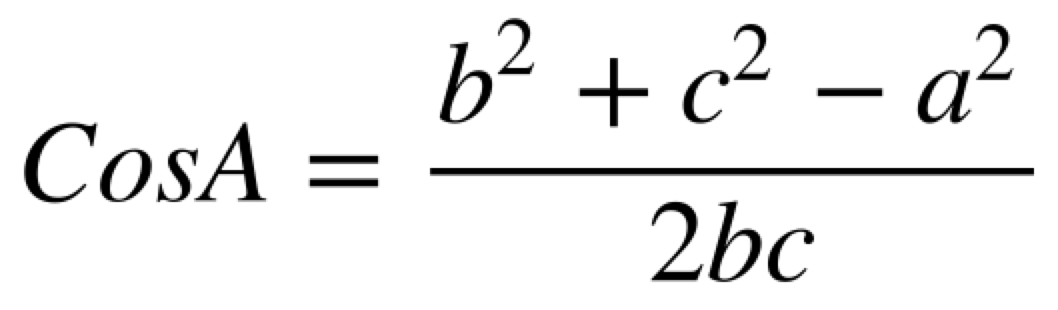July 27, 2016

There may be alternative correct solutions to the ones provided below.

Workout

Question 1

(a) x = 2A – y

(b)(c)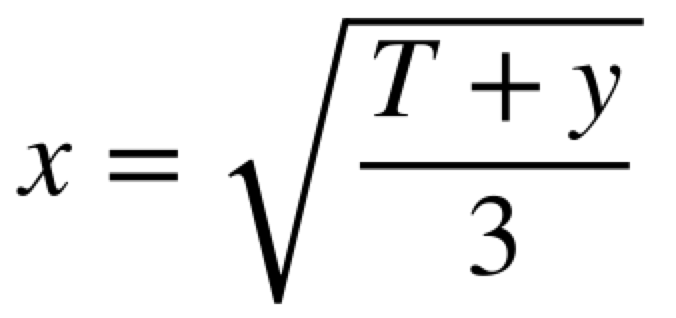(d)(e)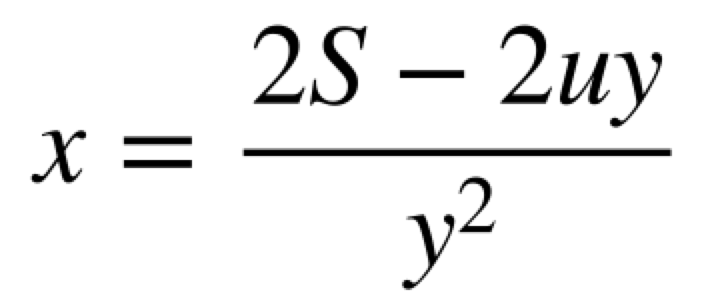(f)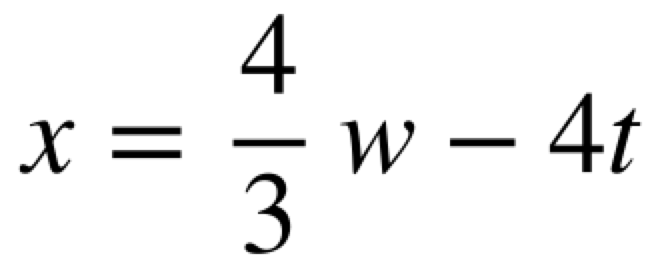(g)  x = dj – 3

(h)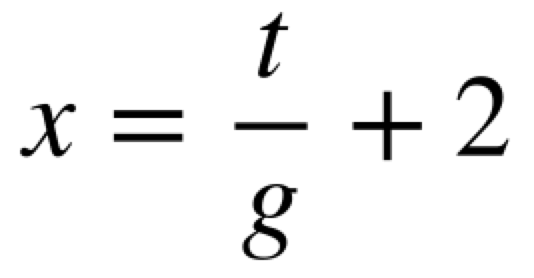(i)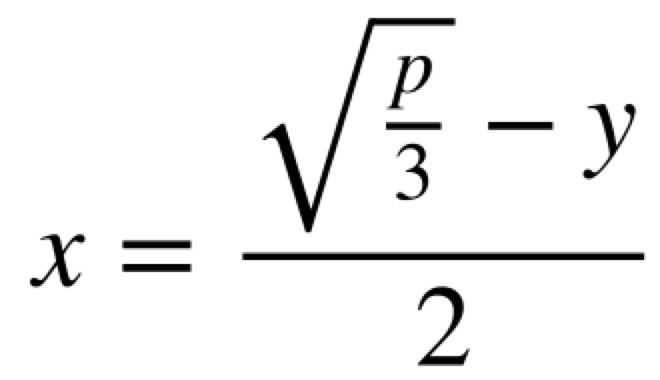(j)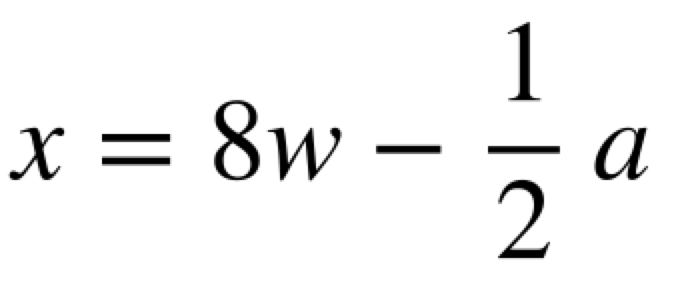Question 2

(a)  m = -17y

(b)  m = 7a – 6

(c)(d)(e)(f)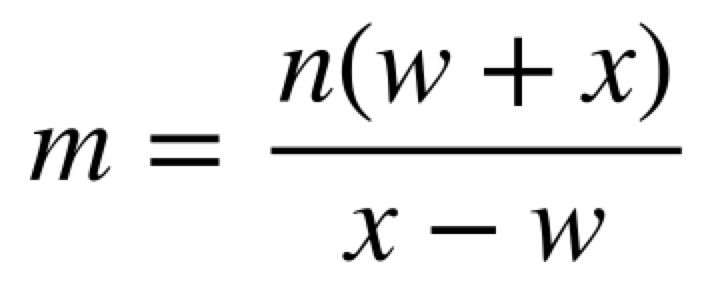(g)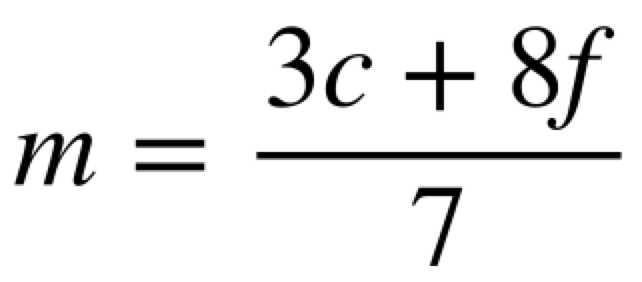(h)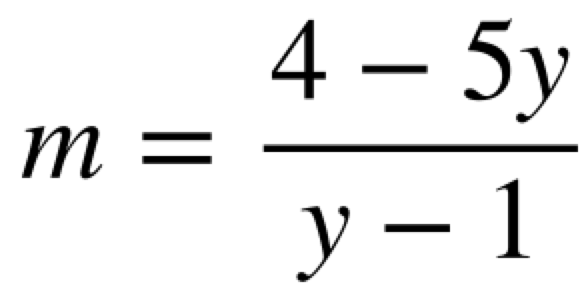(i)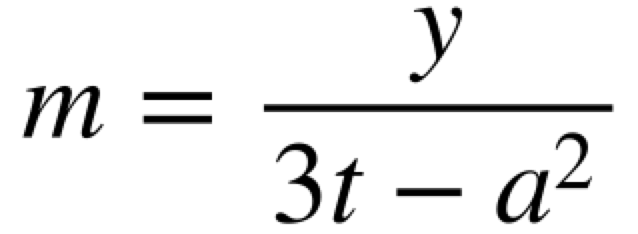(j)(k)(l)    m = k + 2t

(m)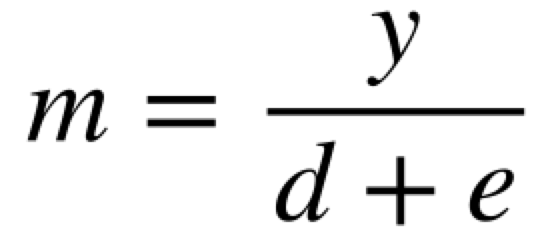(n)(o)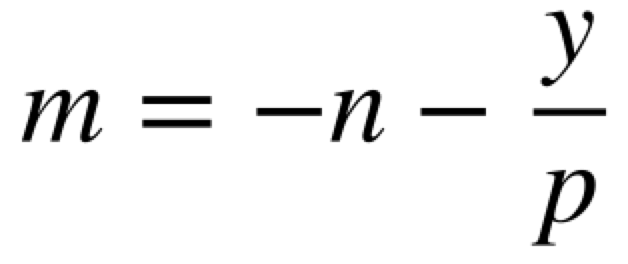(p)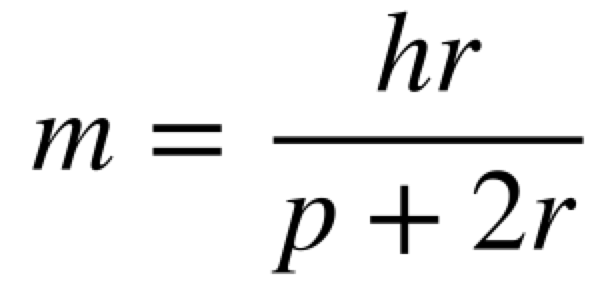(q)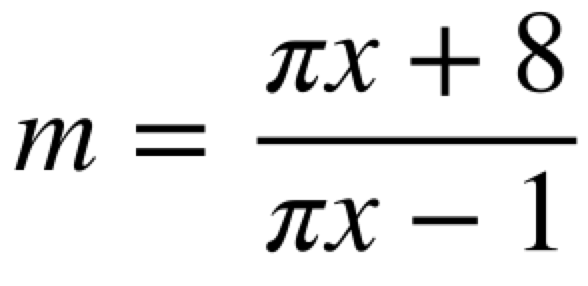(r)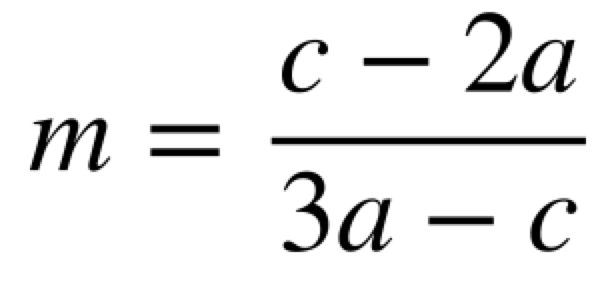Question 3

(a)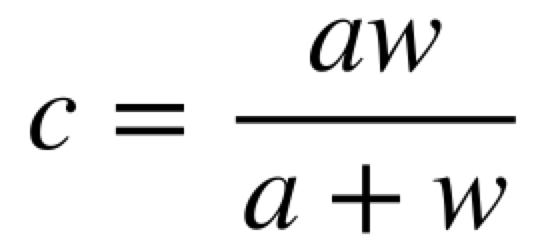(b)Apply

Question 1: